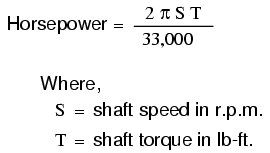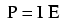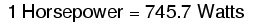# Power in electric circuits

In addition to voltage and current, there is another measure of free electron activity in a circuit: power. First, we need to understand just what power is before we analyze it in any circuits.

Power is a measure of how much work can be performed in a given amount of time. Work is generally defined in terms of the lifting of a weight against the pull of gravity. The heavier the weight and/or the higher it is lifted, the more work has been done. Power is a measure of how rapidly a standard amount of work is done.

For American automobiles, engine power is rated in a unit called “horsepower,” invented initially as a way for steam engine manufacturers to quantify the working ability of their machines in terms of the most common power source of their day: horses. One horsepower is defined in British units as 550 ft-lbs of work per second of time. The power of a car’s engine won’t indicate how tall of a hill it can climb or how much weight it can tow, but it will indicate how fast it can climb a specific hill or tow a specific weight.

The power of a mechanical engine is a function of both the engine’s speed and its torque provided at the output shaft. Speed of an engine’s output shaft is measured in revolutions per minute, or RPM. Torque is the amount of twisting force produced by the engine, and it is usually measured in pound-feet, or lb-ft (not to be confused with foot-pounds or ft-lbs, which is the unit for work). Neither speed nor torque alone is a measure of an engine’s power.

A 100 horsepower diesel tractor engine will turn relatively slowly, but provide great amounts of torque. A 100 horsepower motorcycle engine will turn very fast, but provide relatively little torque. Both will produce 100 horsepower, but at different speeds and different torques. The equaton for shaft horsepower is simple:Notice how there are only two variable terms on the right-hand side of the equation, S and T. All the other terms on that side are constant: 2, pi, and 33,000 are all constants (they do not change in value). The horsepower varies only with changes in speed and torque, nothing else. We can re-write the equation to show this relationship:Because the unit of the “horsepower” doesn’t coincide exactly with speed in revolutions per minute multiplied by torque in pound-feet, we can’t say that horsepower equals ST. However, they are proportional to one another. As the mathematical product of ST changes, the value for horsepower will change by the same proportion.

In electric circuits, power is a function of both voltage and current. Not surprisingly, this relationship bears striking resemblance to the “proportional” horsepower formula above:In this case, however, power (P) is exactly equal to current (I) multiplied by voltage (E), rather than merely being proportional to IE. When using this formula, the unit of measurement for power is the watt, abbreviated with the letter “W.”

It must be understood that neither voltage nor current by themselves constitute power. Rather, power is the combination of both voltage and current in a circuit. Remember that voltage is the specific work (or potential energy) per unit charge, while current is the rate at which electric charges move through a conductor. Voltage (specific work) is analogous to the work done in lifting a weight against the pull of gravity. Current (rate) is analogous to the speed at which that weight is lifted. Together as a product (multiplication), voltage (work) and current (rate) constitute power.

Just as in the case of the diesel tractor engine and the motorcycle engine, a circuit with high voltage and low current may be dissipating the same amount of power as a circuit with low voltage and high current. Neither the amount of voltage alone nor the amount of current alone indicates the amount of power in an electric circuit.

In an open circuit, where voltage is present between the terminals of the source and there is zero current, there is zero power dissipated, no matter how great that voltage may be. Since P=IE and I=0 and anything multiplied by zero is zero, the power dissipated in any open circuit must be zero. Likewise, if we were to have a short circuit constructed of a loop of superconducting wire (absolutely zero resistance), we could have a condition of current in the loop with zero voltage, and likewise no power would be dissipated. Since P=IE and E=0 and anything multiplied by zero is zero, the power dissipated in a superconducting loop must be zero. (We’ll be exploring the topic of superconductivity in a later chapter).

Whether we measure power in the unit of “horsepower” or the unit of “watt,” we’re still talking about the same thing: how much work can be done in a given amount of time. The two units are not numerically equal, but they express the same kind of thing. In fact, European automobile manufacturers typically advertise their engine power in terms of kilowatts (kW), or thousands of watts, instead of horsepower! These two units of power are related to each other by a simple conversion formula:So, our 100 horsepower diesel and motorcycle engines could also be rated as “74570 watt” engines, or more properly, as “74.57 kilowatt” engines. In European engineering specifications, this rating would be the norm rather than the exception.

• REVIEW:
• Power is the measure of how much work can be done in a given amount of time.
• Mechanical power is commonly measured (in America) in “horsepower.”
• Electrical power is almost always measured in “watts,” and it can be calculated by the formula P = IE.
• Electrical power is a product of both voltage and current, not either one separately.
• Horsepower and watts are merely two different units for describing the same kind of physical measurement, with 1 horsepower equaling 745.7 watts.

## Calculating electric power

We’ve seen the formula for determining the power in an electric circuit: by multiplying the voltage in “volts” by the current in “amps” we arrive at an answer in “watts.” Let’s apply this to a circuit example:In the above circuit, we know we have a battery voltage of 18 volts and a lamp resistance of 3 Ω. Using Ohm’s Law to determine current, we get:Now that we know the current, we can take that value and multiply it by the voltage to determine power:Answer: the lamp is dissipating (releasing) 108 watts of power, most likely in the form of both light and heat.

Let’s try taking that same circuit and increasing the battery voltage to see what happens. Intuition should tell us that the circuit current will increase as the voltage increases and the lamp resistance stays the same. Likewise, the power will increase as well:Now, the battery voltage is 36 volts instead of 18 volts. The lamp is still providing 3 Ω of electrical resistance to the flow of electrons. The current is now:This stands to reason: if I = E/R, and we double E while R stays the same, the current should double. Indeed, it has: we now have 12 amps of current instead of 6. Now, what about power?Notice that the power has increased just as we might have suspected, but it increased quite a bit more than the current. Why is this? Because power is a function of voltage multiplied by current, and both voltage and current doubled from their previous values, the power will increase by a factor of 2 x 2, or 4. You can check this by dividing 432 watts by 108 watts and seeing that the ratio between them is indeed 4.

Using algebra again to manipulate the formulae, we can take our original power formula and modify it for applications where we don’t know both voltage and current:

If we only know voltage (E) and resistance (R):If we only know current (I) and resistance (R):An historical note: it was James Prescott Joule, not Georg Simon Ohm, who first discovered the mathematical relationship between power dissipation and current through a resistance. This discovery, published in 1841, followed the form of the last equation (P = I2R), and is properly known as Joule’s Law. However, these power equations are so commonly associated with the Ohm’s Law equations relating voltage, current, and resistance (E=IR ; I=E/R ; and R=E/I) that they are frequently credited to Ohm.• REVIEW:
• Power measured in watts, symbolized by the letter “W”.
• Joule’s Law: P = I2R ; P = IE ; P = E2/R

### Related Posts:

COMMENT songs

CONTACT
FAVORITE
SITEMAP
VLSI COMPANIES IN BANGALORE
VLSI INTERVIEW QUESTION
RFSIR.COM